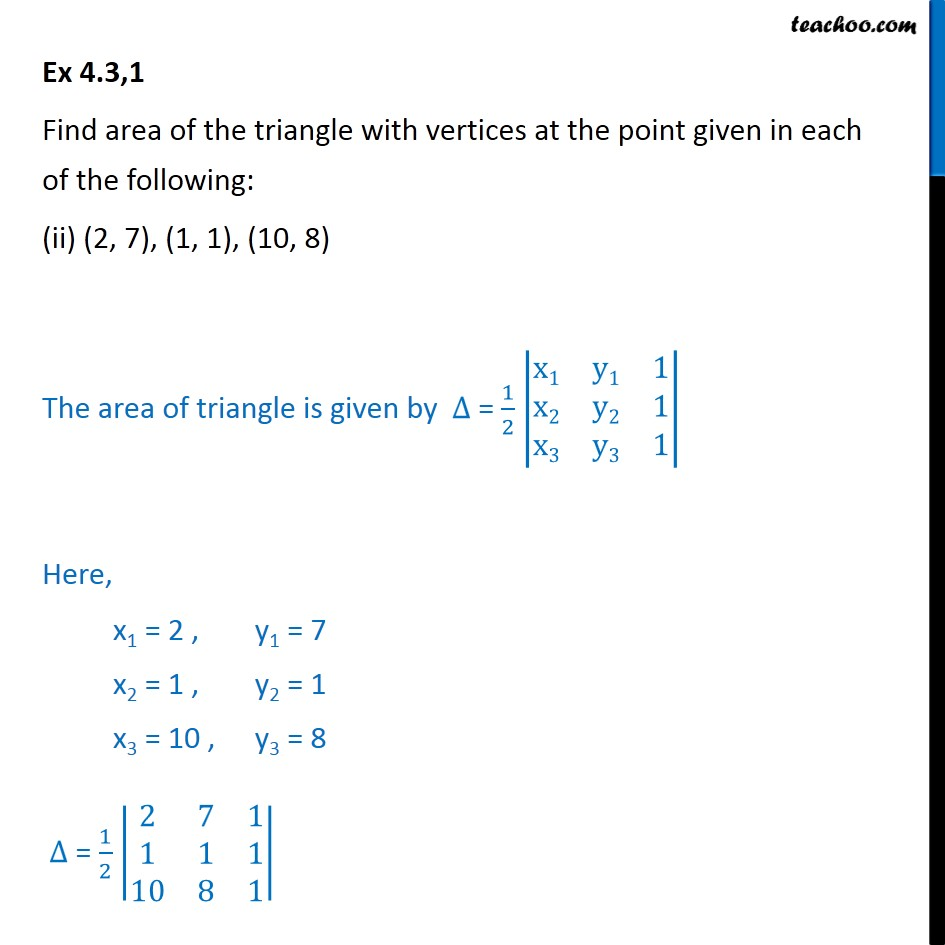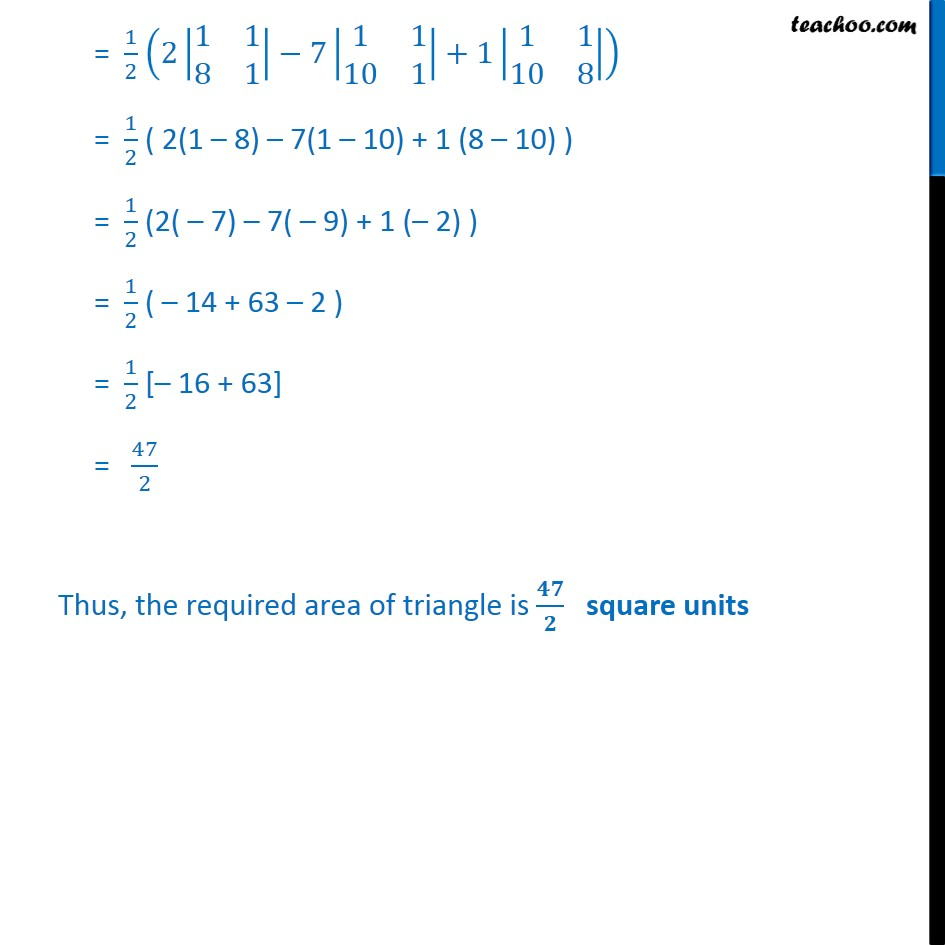Ex 4.2

Chapter 4 Class 12 Determinants
Serial order wiseLearn in your speed, with individual attention - Teachoo Maths 1-on-1 Class

### Transcript

Ex 4.2,1 Find area of the triangle with vertices at the point given in each of the following: (ii) (2, 7), (1, 1), (10, 8) The area of triangle is given by ∆ = 1﷮2﷯ x1﷮y1﷮1﷮x2﷮y2﷮1﷮x3﷮y3﷮1﷯﷯ Here, x1 = 2 , y1 = 7 x2 = 1 , y2 = 1 x3 = 10 , y3 = 8 ∆ = 1﷮2﷯ 2﷮7﷮1﷮1﷮1﷮1﷮10﷮8﷮1﷯﷯ = 1﷮2﷯ 2 1﷮1﷮8﷮1﷯﷯−7 1﷮1﷮10﷮1﷯﷯+1 1﷮1﷮10﷮8﷯﷯﷯ = 1﷮2﷯ ( 2(1 – 8) – 7(1 – 10) + 1 (8 – 10) ) = 1﷮2﷯ (2( – 7) – 7( – 9) + 1 (– 2) ) = 1﷮2﷯ ( – 14 + 63 – 2 ) = 1﷮2﷯ [– 16 + 63] = 47﷮2﷯ Thus, the required area of triangle is 𝟒𝟕﷮𝟐﷯ square units